##### Multicollinearity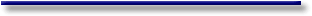# Online Econometrics Textbook - Regression Extensions - Multicollinearity - Remedies to the multicollinearity problem

#### III.IV.2 Remedies to the multicollinearity problem

Let us have a brief look at some possible solutions that may be used to solve the harmful effects of the multicollinearity problem.

##### 1. drop spurious exogenous variables

Assume we were interested in the estimation of the model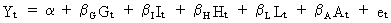(III.IV.2-1)

where G, I, H, L, and A are exogenous variables.

Suppose that harmful multicollinearity would have been discovered between G, I, and H and between L and A. Then we may chose one representative of each group (e.g. G and L). All the other exogenous variables may be dropped since they do not entail any information which is not present in either G or L.

##### 2. principal components

As we have seen before, X'X can be diagonalized and written in terms of eigenvectors and eigenvalues. Accordingly, the linear model can be written in terms of its principal components (see (III.IV-4)). The first principal component can intuitively be interpreted as the summary of all exogenous variables by one column vector which explains as much of X as possible. The remaining information is entailed in the second principal component and so on ... It is however important to note that the principal components are orthogonal and therefore cannot be multicollinear.

Suppose we would have computed the principal components for our model of (III.IV.2-1). Also assume that the principal components (PC) contain (in descending order) 90%, 5%, 4%, ... of the total variance of the exogenous variables. In such circumstances we would retain the first three PC in our regression model since they account for 99% of the variance of X.

When having three PCs in a regression model, this means that there are three important groups of variables (within the set of X) which are explaining the endogenous variable. Cross correlations between the exogenous variables and the PC should reveal which variables may be associated with different factors (this is necessary for interpretation purposes).

Now suppose that this regression would result in only the first PC to be significantly different from zero. In this case our model would reduce to a simple regression. The only problem with this is that we have no clue of how this model should be interpreted, since one PC cannot directly be assigned to a specific exogenous variable (but rather to a combination of all variables).

Therefore, in such circumstances, it could be better to compute the PC for both subgroups that we have detected before. We may present the X matrix as follows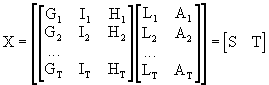(III.IV.2-2)

and compute the PC for S and T separately. This process will probably result in at least one significant PC-parameter per subgroup in a multiple regression with the endogenous, and therefore it is possible to interpret the model easily. Note however that in this case there is no reason to assume automatically that the first PC of S and the first PC or T are not multicollinear (since both PCs have been computed separately, and since our detection of, and splitting the variables into two subgroups, might have been wrong).

##### 3. ridge regression

The estimator for ridge regression is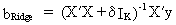(III.IV.2-3)

where delta is a small number which is to be added to the diagonal elements of X'X. Be aware of the fact that there exists a sensitivity of the parameters with respect to the ridge parameter delta (therefore several values for delta might be attempted before deciding upon the final ridge estimation results).

##### 4. first differences

The first differences of a time series are defined by(III.IV.2-4)

A disadvantage of this differencing is obviously the loss of one degree of freedom since the series becomes shorter. Also note that this differencing is exclusively used with time series (and has mostly no relevance with cross-section data).

The relevance and interpretation will be comprehensively clarified in chapter V (time series analysis).

The only relevant thing to remember now, is that differencing alters the time series so that it can be seen as the change of the series. For instance the model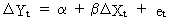(III.IV.2-5)

illustrates the effect of the change of Xt on the change of Yt.

When a time series is differenced twice, it is not interpreted as the absolute change but rather as the acceleration of the series.

##### 5). ratio's and deflating series

It is sometimes useful to use the ratio's of two (or more) multicollinear series. In our example we could for instance redefine the exogenous variables as

rgi = G / I
rhi = H / I
rla = L / A

which doesn't reduce the degrees of freedom, and maintains all variables in the model. Though, care should be taken with respect to the interpretation of the estimated parameters.

Another common remedy to the multicollinearity problem is deflating time series (mostly prices, or price indexes) by some time series measuring e.g. consumption prices. Thus, in stead of working with nominal quantities it is preferred to use real quantities.

##### 6). additional information and restrictions

Sometimes economists have additional, or a priori information about the model. This information could be in the form of knowledge about the true value of some parameter, knowledge about an upper or lower bound for parameters, or knowledge about dependencies between the sensitivity parameters of different exogenous variables.

Such information could be introduced into the model using Restricted Least Squares (RLS) or Restricted MLE (RMLE). For the moment, abstraction is made of Bayesian methods where restrictions can be imposed stochastically in stead of deterministically (see also chapter V).© 2000-2022 All rights reserved. All Photographs (jpg files) are the property of Corel Corporation, Microsoft and their licensors. We acquired a non-transferable license to use these pictures in this website.
The free use of the scientific content in this website is granted for non commercial use only. In any case, the source (url) should always be clearly displayed. Under no circumstances are you allowed to reproduce, copy or redistribute the design, layout, or any content of this website (for commercial use) including any materials contained herein without the express written permission.

Information provided on this web site is provided "AS IS" without warranty of any kind, either express or implied, including, without limitation, warranties of merchantability, fitness for a particular purpose, and noninfringement. We use reasonable efforts to include accurate and timely information and periodically updates the information without notice. However, we make no warranties or representations as to the accuracy or completeness of such information, and it assumes no liability or responsibility for errors or omissions in the content of this web site. Your use of this web site is AT YOUR OWN RISK. Under no circumstances and under no legal theory shall we be liable to you or any other person for any direct, indirect, special, incidental, exemplary, or consequential damages arising from your access to, or use of, this web site.

Contributions and Scientific Research: Prof. Dr. E. Borghers, Prof. Dr. P. Wessa
Please, cite this website when used in publications: Xycoon (or Authors), Statistics - Econometrics - Forecasting (Title), Office for Research Development and Education (Publisher), http://www.xycoon.com/ (URL), (access or printout date).